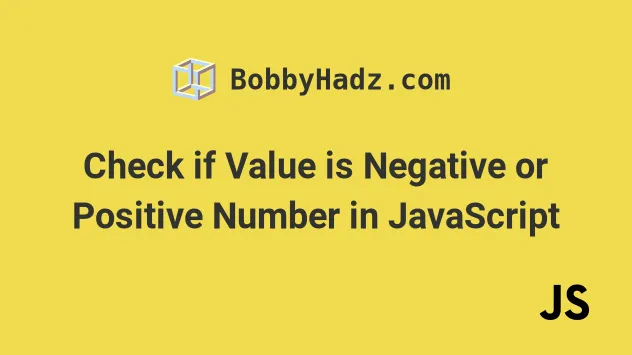# Check if Value is a Negative Number in JavaScriptSun Oct 17 20213 min read## Check if Value is a Negative Number#

To check if a value is a negative number, call the `Math.sign()` method, passing it the value as a parameter. The `Math.sign` method returns `-1` if the provided argument is a negative number or can be converted to one.

index.js
```Copied!```function isNegative(num) {
if (Math.sign(num) === -1) {
return true;
}

return false;
}

console.log(isNegative(-5)); // 👉️ true
console.log(isNegative(5)); // 👉️ false
console.log(isNegative('-5')); // 👉️ true
console.log(isNegative('test')); // 👉️ false
``````

In the code snippet, we use the Math.sign method to check if a value is a negative number.

The only argument the method takes is a `number`. If the provided value is not a number, it gets converted to a number.

If the `Math.sign` method returns `-1`, then the provided argument is a negative number or could be converted to one.

If you want to make sure the passed in parameter is of type `number`, you can add an additional check to the `if` statement.

index.js
```Copied!```function isNegative(num) {
if (typeof num === 'number' && Math.sign(num) === -1) {
return true;
}

return false;
}
``````

We use the logical and (&&) operator to chain another condition. Before calling the `Math.sign` method we check if the provided argument is a number.

The `Math.sign` method has 5 possible return values:

• it returns `1` if the argument is positive
• it returns `-1` if the argument is negative
• it returns `0` if the argument is `0`
• it returns `-0` if the argument is `-0`
• in all other cases it returns `NaN` (not a number)

Here are some examples of calling the `Math.sign` method directly.

index.js
```Copied!```console.log(Math.sign(-5)); // 👉️ -1
console.log(Math.sign(5)); // 👉️ 1
console.log(Math.sign('-5')); // 👉️ -1
console.log(Math.sign('test')); // 👉️ NaN
console.log(Math.sign(0)); // 👉️ 0
``````

If the provided value is not a number, the method attempts to convert it to one.

The `Math.sign` method is not supported in Internet Explorer. If you need to support the browser, use the next approach instead.

To check if a value is a negative number, compare it to `0`, e.g. `num < 0`. If the value to the left hand side of the less-than operator is not already a number, it will get converted to one and compared to `0`. If the expression returns `true`, the value is a negative number.

index.js
```Copied!```// Supported in IE

function isNegative(num) {
if (num < 0) {
return true;
}

return false;
}

console.log(isNegative(-5)); // 👉️ true
console.log(isNegative(5)); // 👉️ false
console.log(isNegative('-5')); // 👉️ true
console.log(isNegative('test')); // 👉️ false
``````

We use the less than (<) operator to compare the value on the left to `0`.

If the value to the left is not a number, JavaScript will attempt to convert it to one.

If you only expect to take numbers as arguments, add another condition to the `if` statement.

index.js
```Copied!```function isNegative(num) {
if (typeof num === 'number' && num < 0) {
return true;
}

return false;
}
``````

Here are some more examples of using the less than operator.

index.js
```Copied!```console.log(-1 < 0); // 👉️ true
console.log('-1' < 0); // 👉️ true
console.log('test' < 0); // 👉️ false
console.log('' < 0); // 👉️ false
console.log(null < 0); // 👉️ false
``````

If you're interested to read more about how the less than operator converts values that are not of the same type when comparing them, check out this section of the MDN docs.